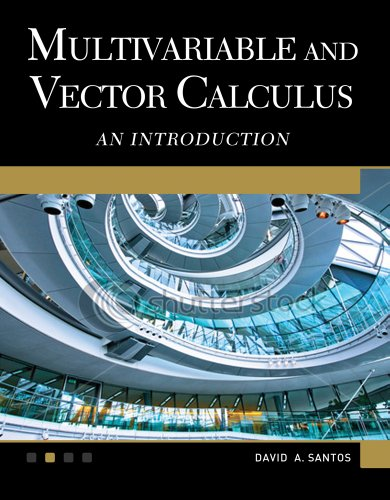# Multivariable and Vector Calculus: An Introduction, 2nd Edition

5.0
Description

This book is designed primarily for undergraduates in mathematics, engineering, and the physical sciences. Rather than concentrating on technical skills, it focuses on a deeper understanding of the subject by providing many unusual and challenging examples. The basic topics of vector geometry, differentiation and integration in several variables are explored. It also provides numerous computer illustrations and tutorials using MATLAB(r) and Maple(r), that bridge the gap between analysis and computation.Features: Includes numerous computer illustrations and tutorials using MATLAB(r) and Maple(r) Covers the major topics of vector geometry, differentiation, and integration in several variables Instructors ancillaries available upon adoption”